# Average Angular Velocity Formula

Average Angular Velocity Formula

The angular velocity of a rotating object is the rate at which the angular coordinate changes with respect to time. The angular coordinate is the angle of the object relative to a certain coordinate system, and is usually represented with the Greek letter θ ("theta"). The average angular velocity is the change in the angular coordinate θ, expressed in radians, divided by the change in time. The angular velocity is a vector that points in the direction of the axis of rotation. The magnitude of the angular velocity is given by the formula below. The unit of angular velocity is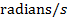.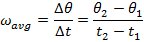= average angular velocity, ()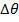= change in angular coordinate (radians)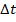= change in time (s)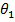= initial angular coordinate (radians)= final angular coordinate (radians)

t1 = initial time (s)

t2 = final time (s)

Average Angular Velocity Formula Questions:

1) The pendulum of a large grandfather clock swings between angles ofand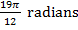. It swings from one angle to the other in 1.00 seconds. What is the average angular velocity of the pendulum as it swings from one angle to the other?

Answer: The change in time between the two angular coordinate values is given as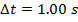. The initial angular coordinate is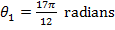, and the final angular coordinate is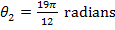. The average angular velocity can be found using the formula: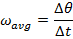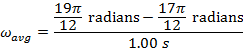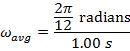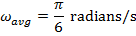Between the initial and final angles, the average angular velocity of the clock's pendulum was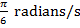, which is approximately.

2) A powerful fan blade spins at an average angular velocity of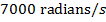. How long does it take, in seconds, to rotate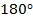?

Answer: The change in the angular coordinate requested in this problem is. The first step to answering this problem is to convert from degrees to radians. A full circle consists of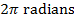, or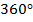. The change in the angular coordinate is therefore: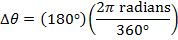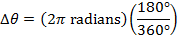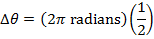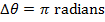The time required for the fan to rotate by this amount can be found by rearranging the average angular velocity formula: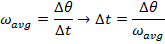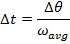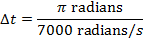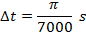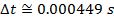The time required for the fan to rotateis only.